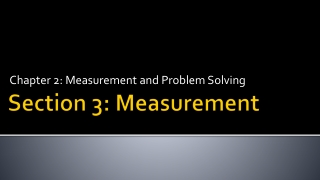Download PresentationSection 3: Measurement

# Section 3: Measurement

Télécharger la présentation## Section 3: Measurement

- - - - - - - - - - - - - - - - - - - - - - - - - - - E N D - - - - - - - - - - - - - - - - - - - - - - - - - - -
##### Presentation Transcript

1. Chapter 2: Measurement and Problem Solving Section 3: Measurement

2. Learning Objectives • Practice measuring according to significant figures.

3. Measurement • What is the volume of water in the graduated cylinder in mL? • How many sigfigs is this? • How many L is this? • Express this number in sci. notation.

4. Measurement • Always take the measurement to one decimal place past the smallest gradation (tic mark) • Graduated cylinders • Beakers/flasks/bottles /etc. are NOT used for measuring! Measure the volume of liquids ONLY with a graduated cylinder • Rulers • When using the electronic balance, record the entire number on the screen

5. 21.75 mL

6. 2.65 cm

7. 3.53 cm

8. Measurement • The bathroom scale has markings at every 1 lb. Report the reading to the correct number of digits.

9. Measurement • The unit system for science measurements, based on the metric system, is called the International System of Units or SI units.

10. Measurement • The standard of length • The definition of a meter, established by international agreement in 1983, is the distance that light travels in vacuum in 1/299,792,458 s. (The speed of light is 299,792,458 m/s.)

11. Measurement • The standard of mass • The kilogram is defined as the mass of a block of metal kept at the International Bureau of Weights and Measures at Sèvres, France. A duplicate is kept at the National Institute of Standards and Technology near Washington, D.C.

12. Measurement • The kilogram is a measure of mass, which is different from weight. • The mass of an object is a measure of the quantity of matter within it. • The weight of an object is a measure of the gravitational pull on that matter. • Consequently, weight depends on gravity while mass does not.

13. Measurement • The standard of time • The second is defined, using an atomic clock, as the duration of 9,192,631,770 periods of the radiation emitted from a certain transition in a cesium-133 atom.